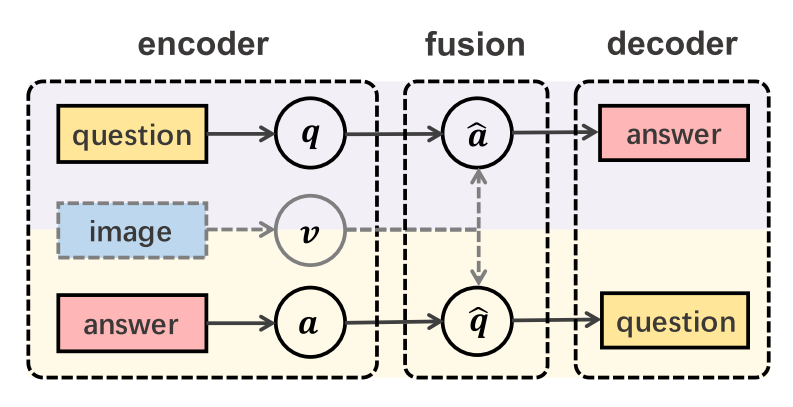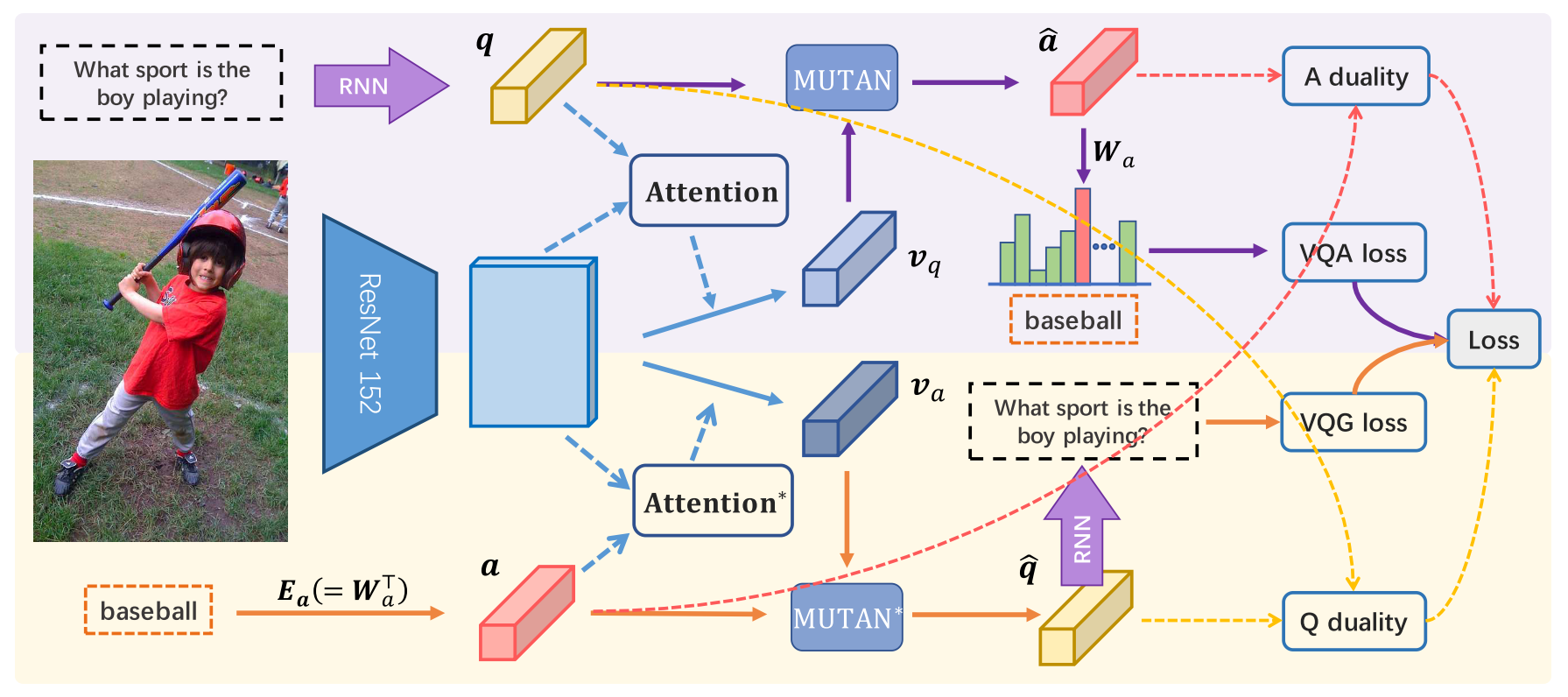## WHY?

Visual question answering and visual question generation are complementary tasks. Learning one task may benefit the other.

## WHAT?This paper suggests Invertible Question Answering Network(iQAN) that is trained on two tasks sharing pipline in reverse order.VQA model of iQAN is MUTAN fusion module, and another MUTAN-based attention module is used for VQG.In order to benefit from the other task, weights are shared between two processes. Dual MUTAN shares core tensor and projection matrices of two MUTAN modules. In addition, input answer projection matrix of VQG model and linear classifier matrix of VQA model are shared. While two RNN for VQG and VQA may not share the parameter, word embedding matrices are shared.

Since two processes are cyclic, duality regularizer is used to promote consistency. Two losses from both tasks(multinomial classification loss for VQA and sequence generation loss for VQG) and two regularizers are jointly trained in dual training manner.

Loss = L_{(VQA)}(a, a*) + L_{(VQG)}(q, q*) + smooth_{L1}(\mathbf{q} - \hat{\mathbf{q}}) + smooth_{L1}(\mathbf{a} - \hat{\mathbf{a}})

Yes/no or number questions are filtered out.

## So?

iQAN showed comparable result in VQA2 and CLEVR dataset.

Powered by aiden